2019-09-02 11:22:23 wangwei19871103 阅读数 123
• ###### 深度学习基础与TensorFlow实践教程

本课程主要介绍了深度学习的基础原理和TensorFlow系统基本使用方法。TensorFlow是目前机器学习、深度学习领域优秀的计算系统之一，本课程将结合实例介绍使用TensorFlow开发机器学习应用的详细方法和步骤，着重讲解了用于图像识别的卷积神经网络和用于自然语言处理的循环神经网络的理论知识及其TensorFlow实现方法，并结合实际场景和例子描述了深度学习技术的应用范围与效果。 所有案例均来自讲师团队工作中的亲身实践，所选案例均是深度学习的经典应用，非常具有代表性。

21447 人正在学习 去看看 AI100讲师

# 深度强化学习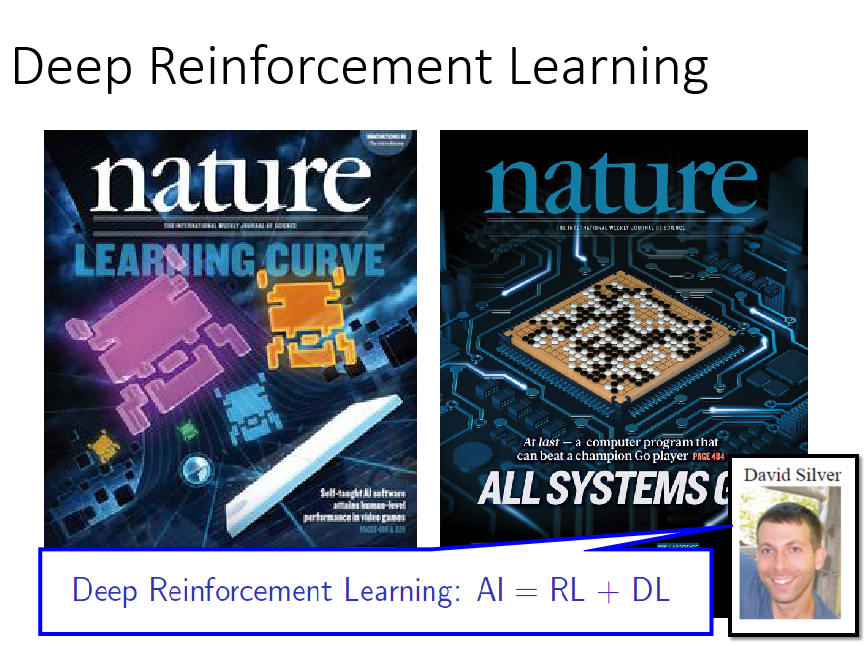# 强化学习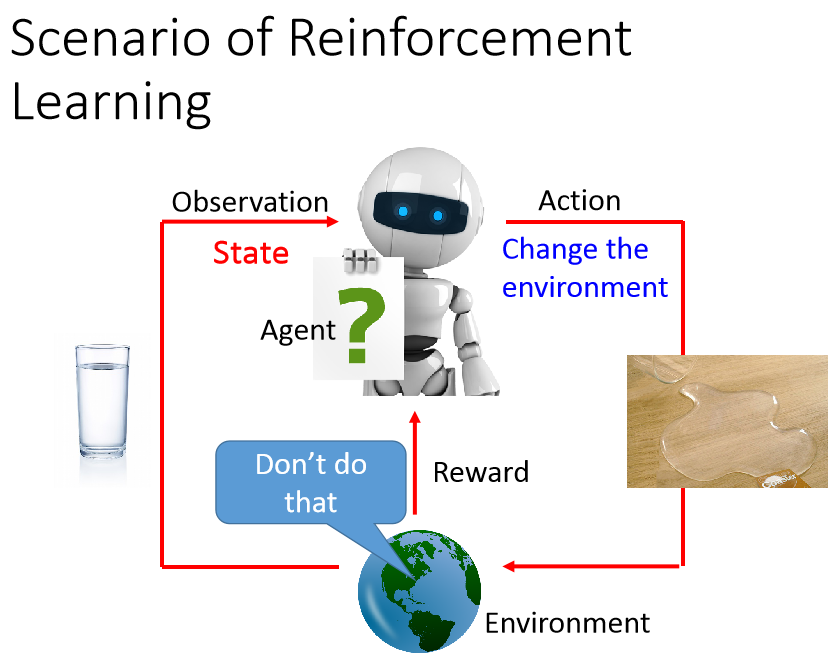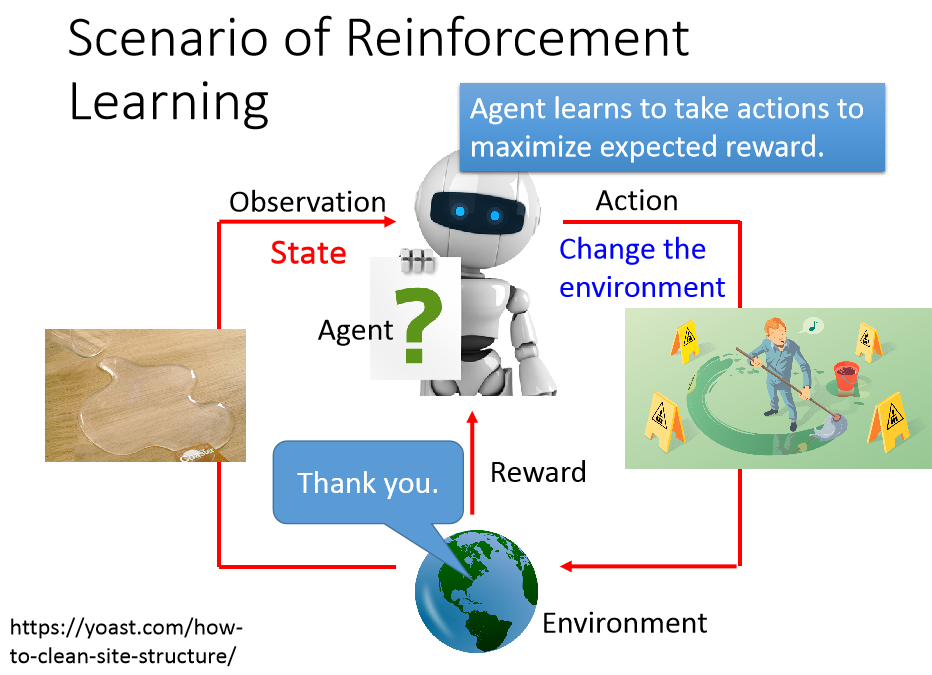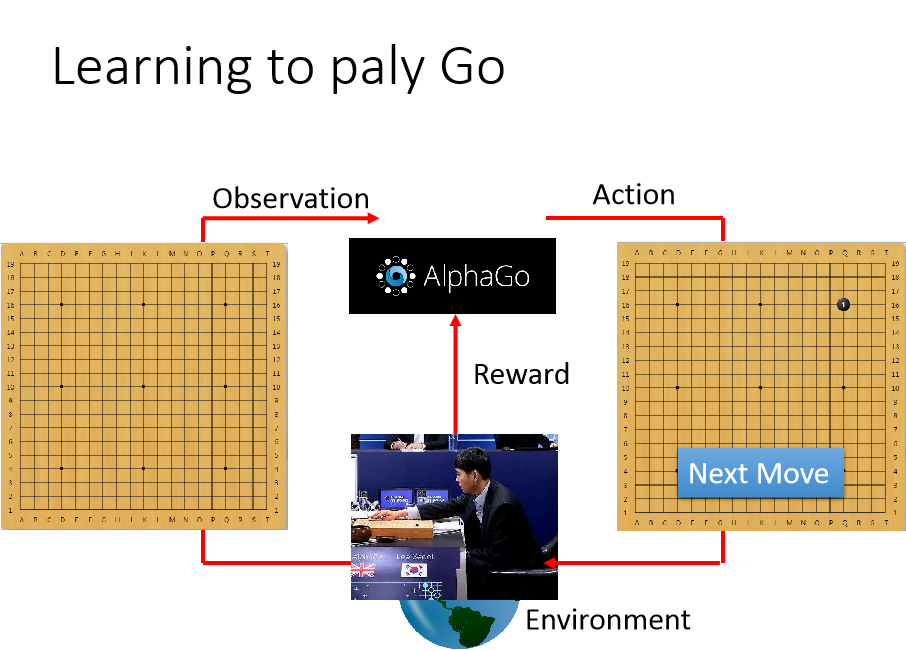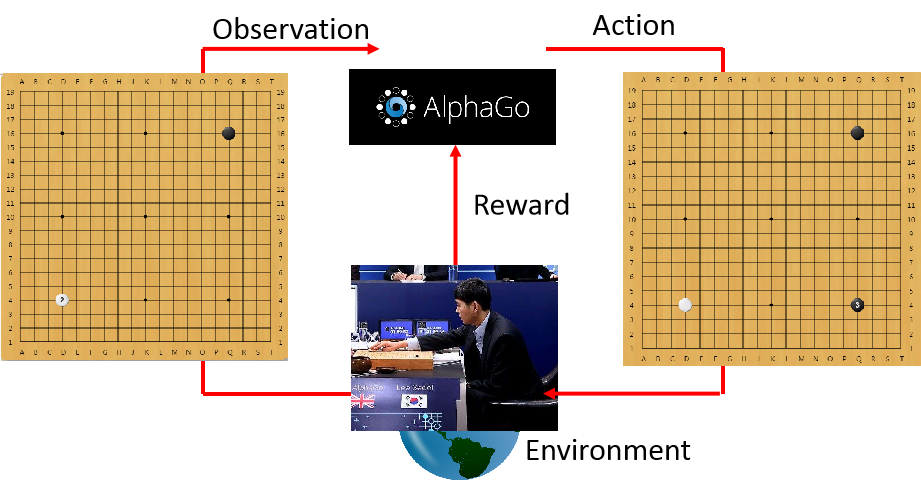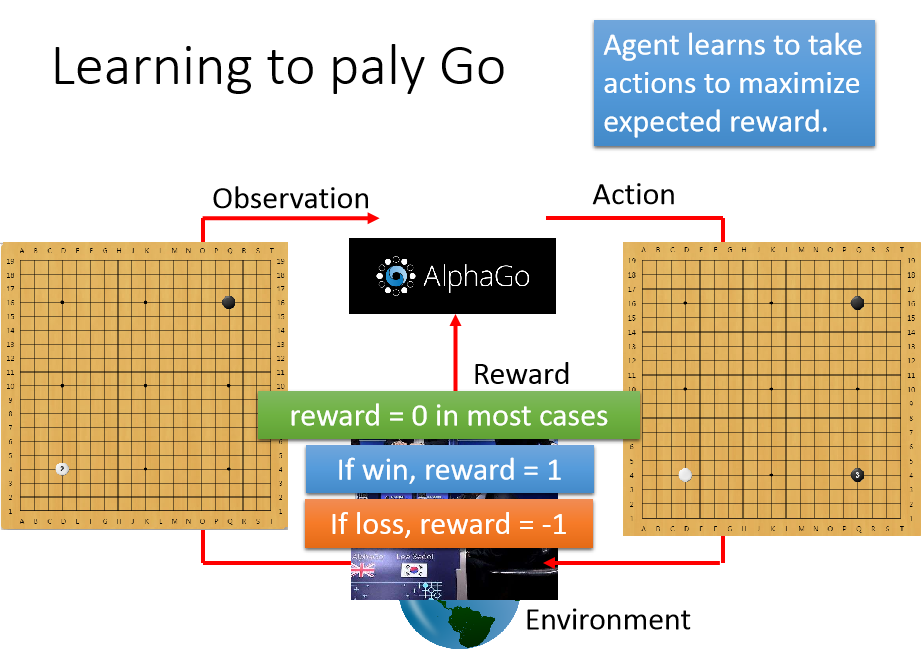## 与监督学习的区别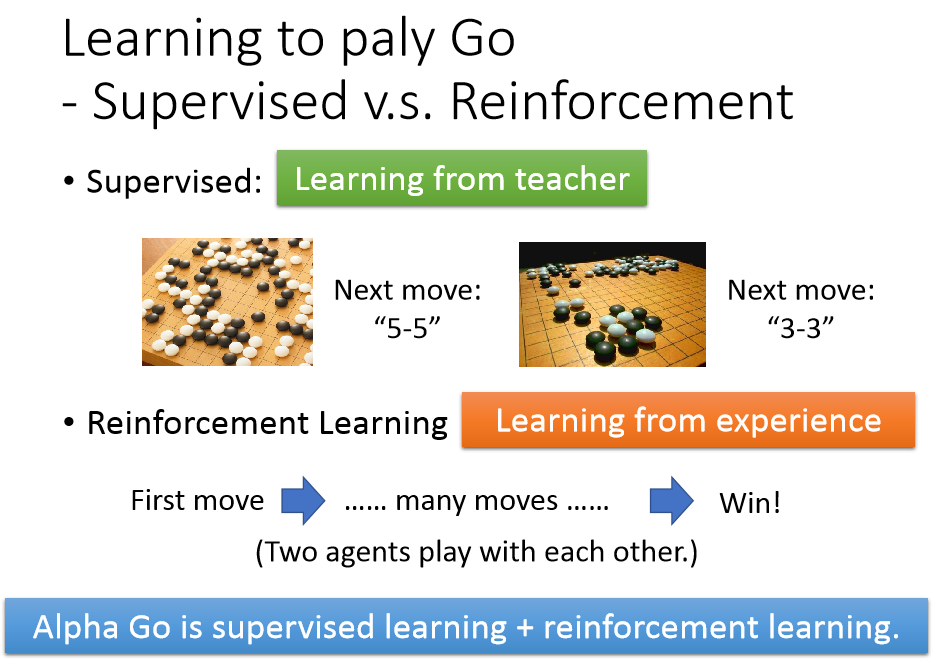## 训练语音对话机器人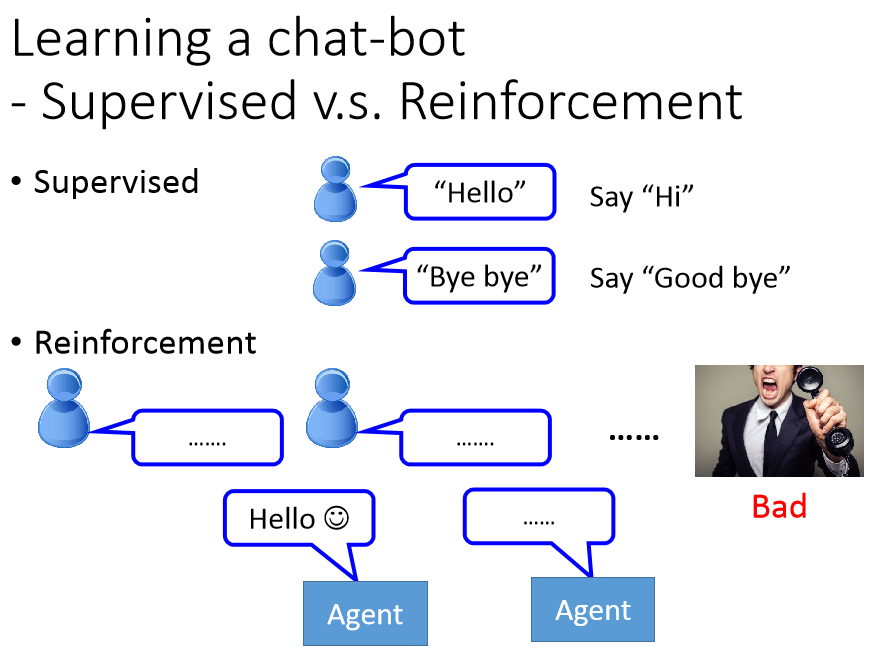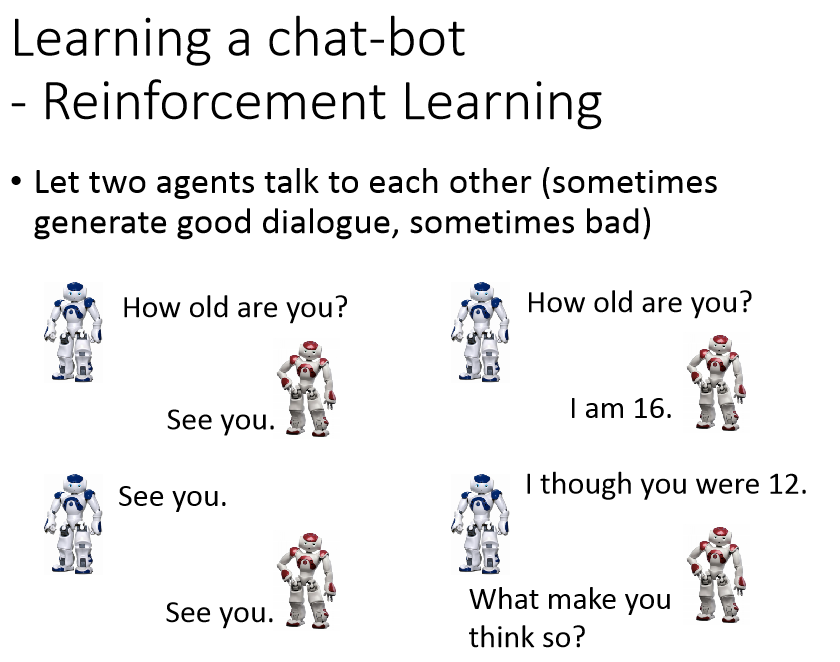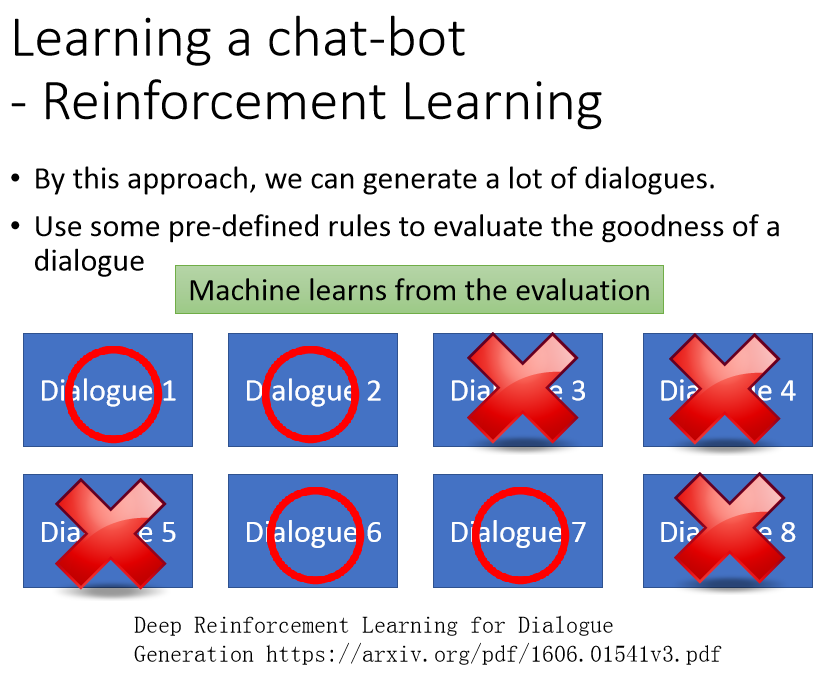## 更多的应用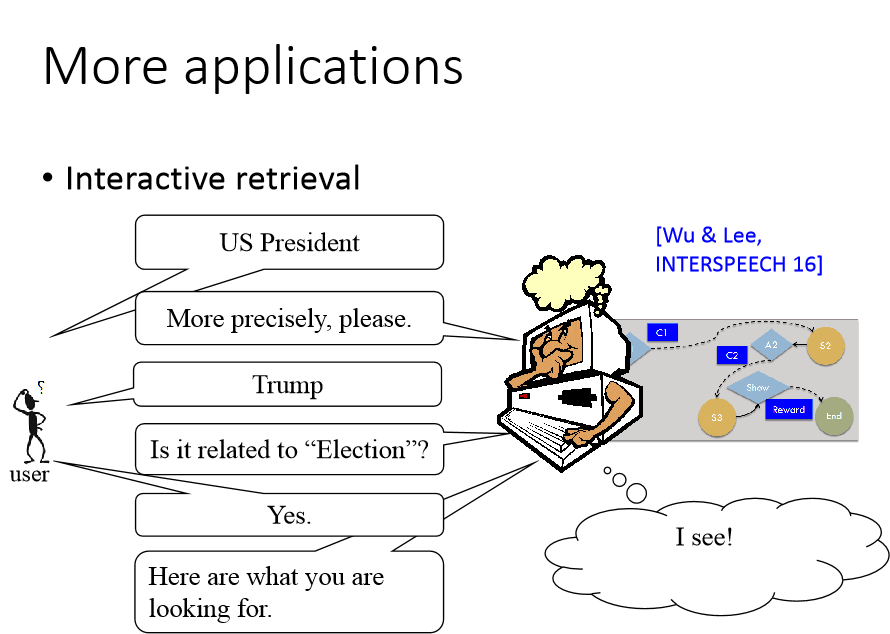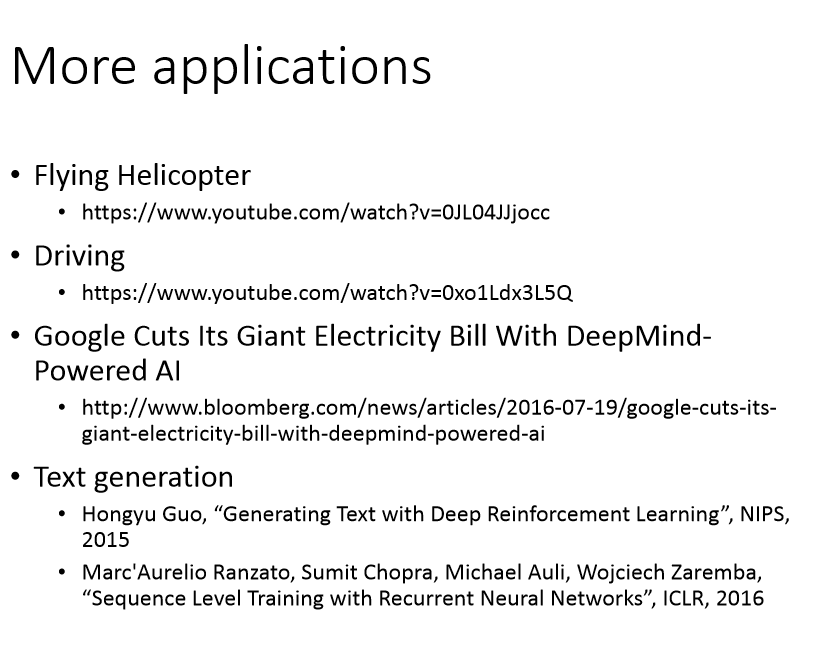## 玩游戏的例子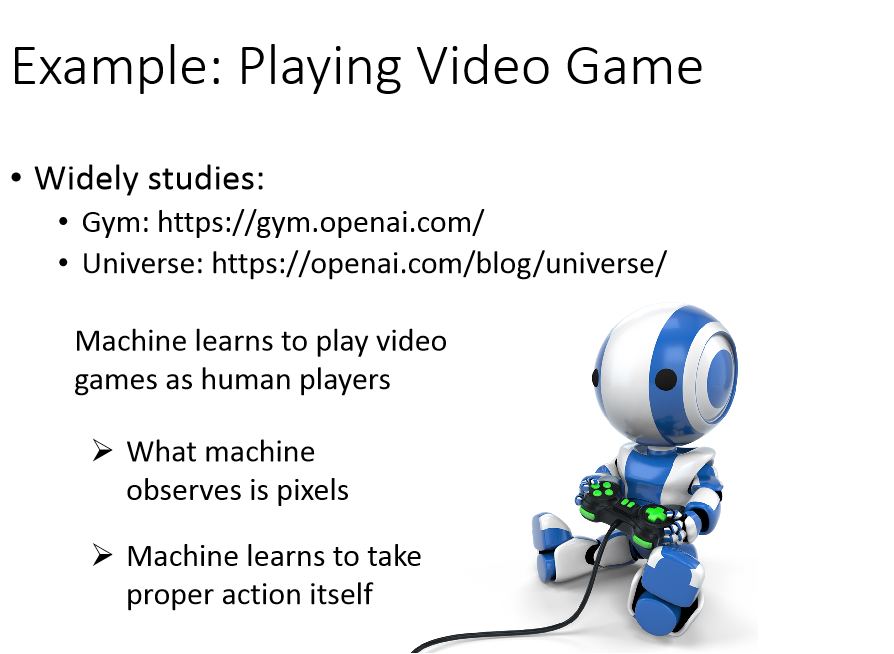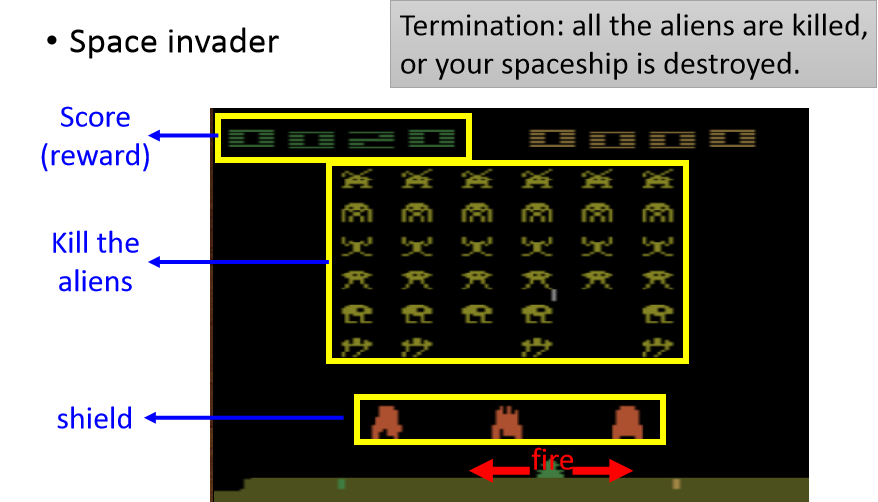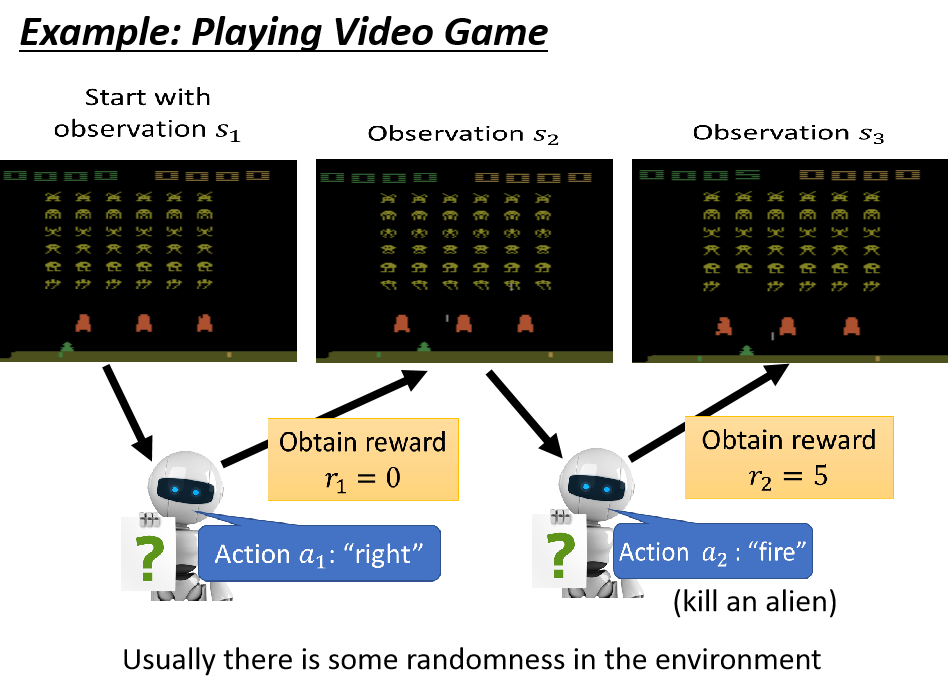如此反复下去直到最后一次采取$a_T$，获得的奖励是$r_T$，游戏结束，这个整个过程我们叫做一个episode，也就是一个开始到结束的一个过程。我们希望最大化机器每次游戏的过程的累计奖励：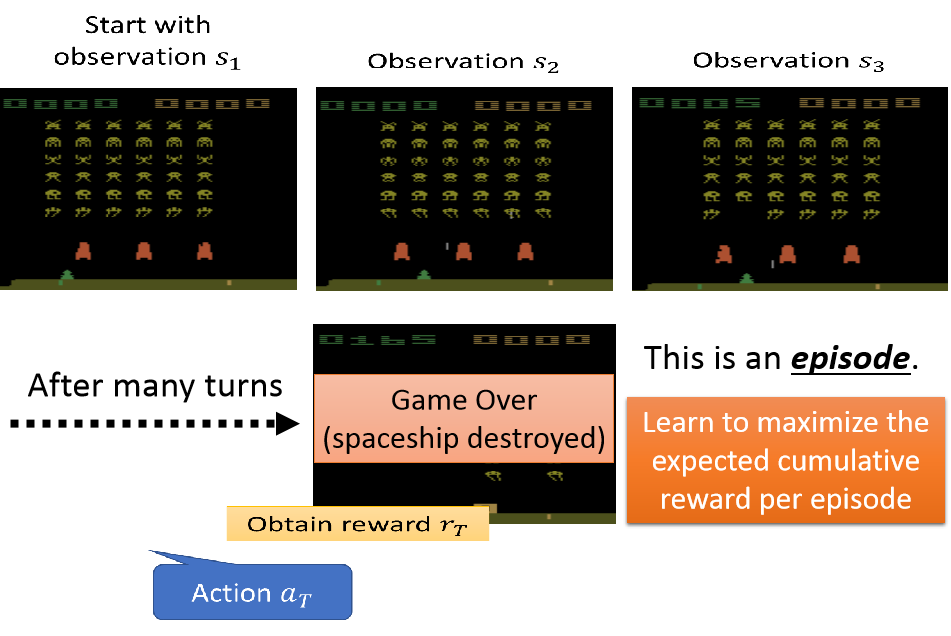## 强化学习的难点：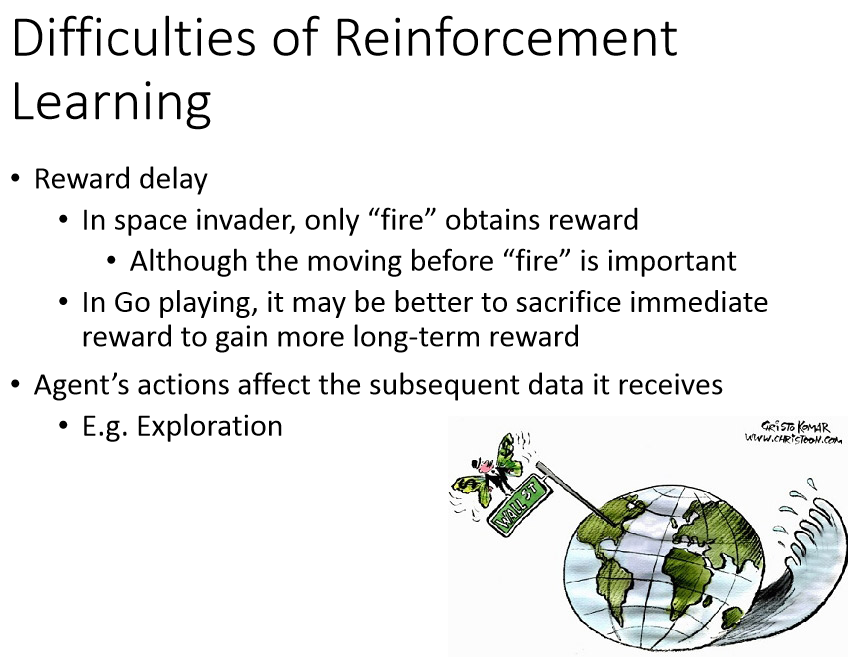## 强化学习比较新的方法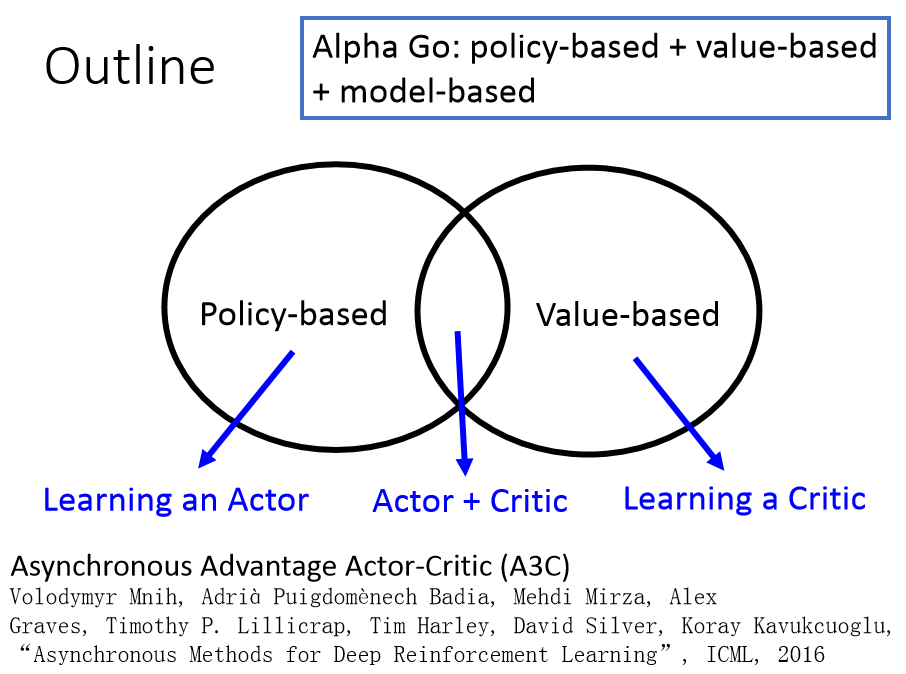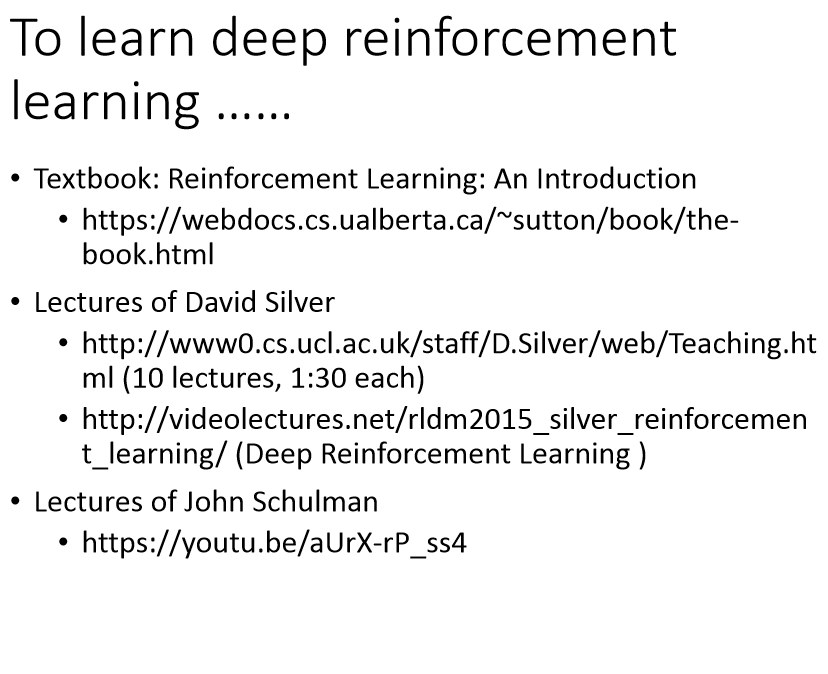## 强化学习基于策略的方法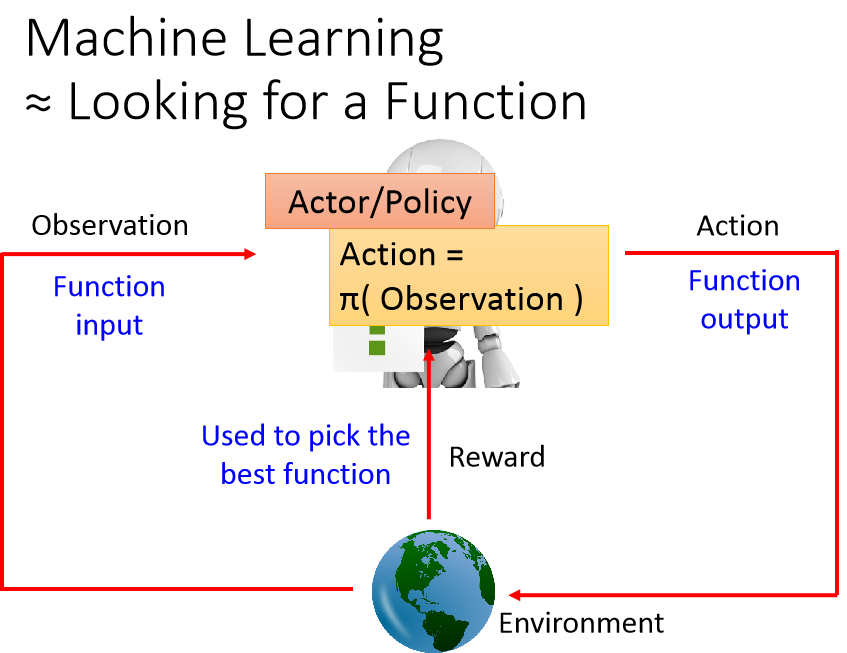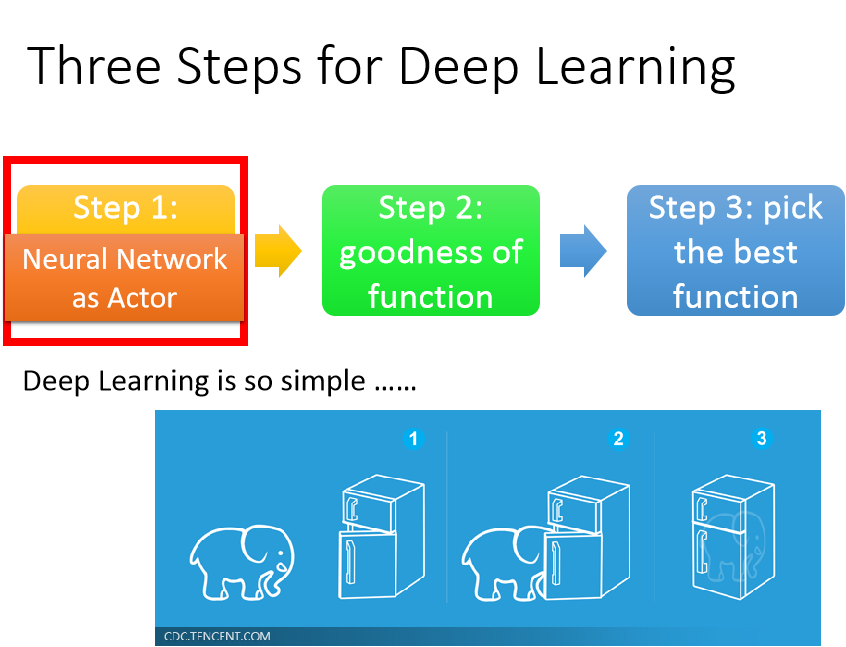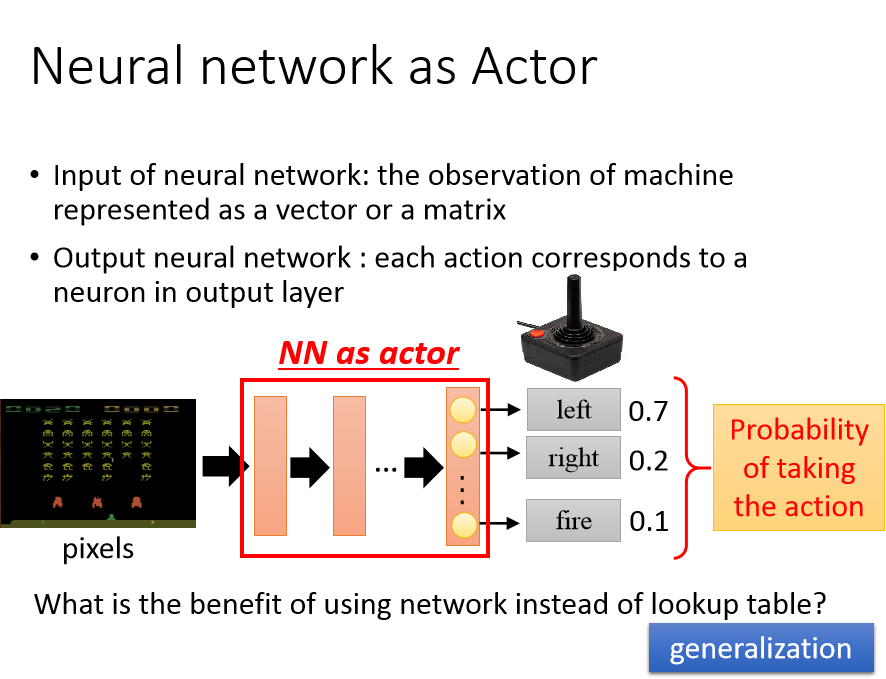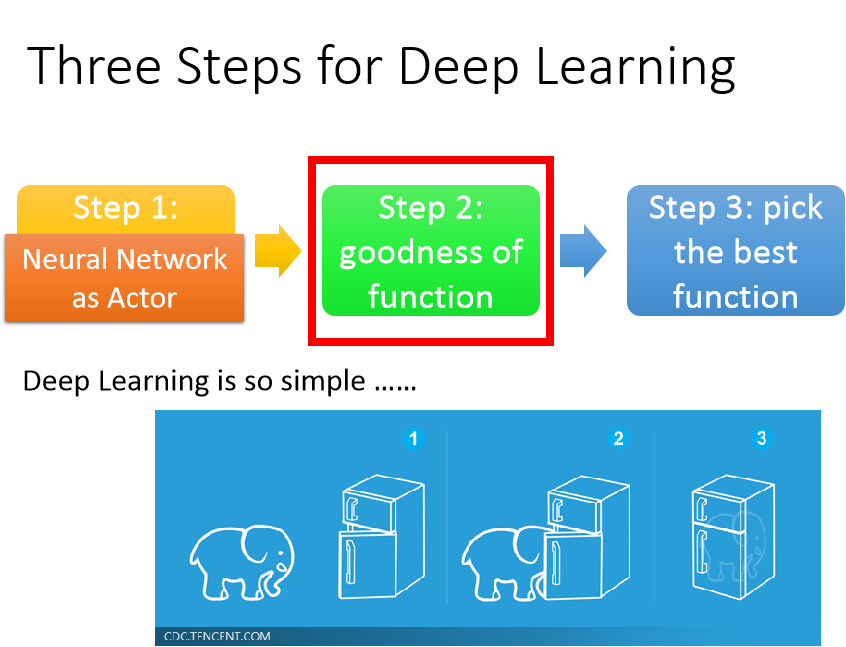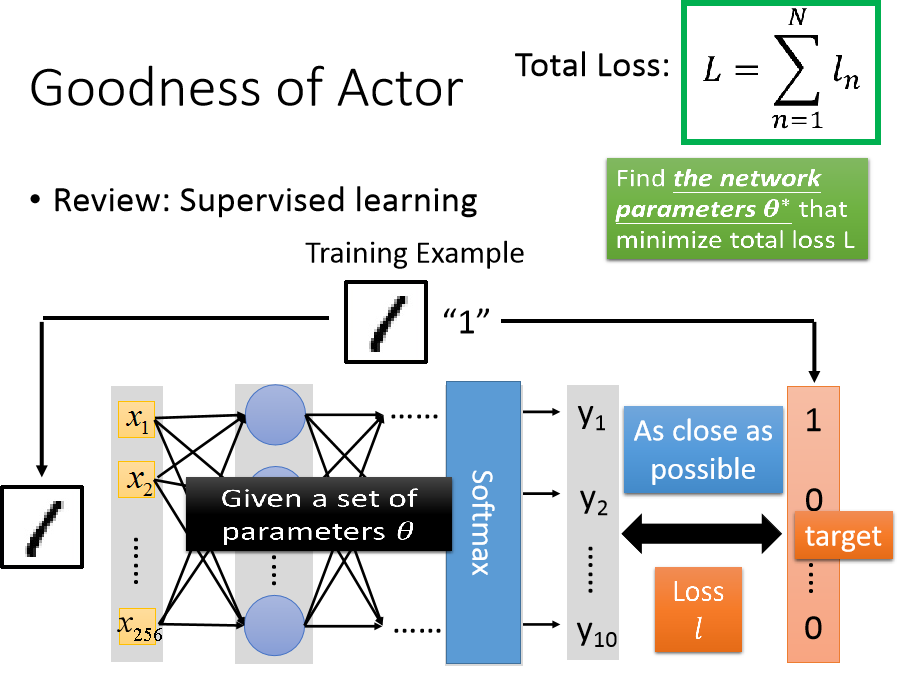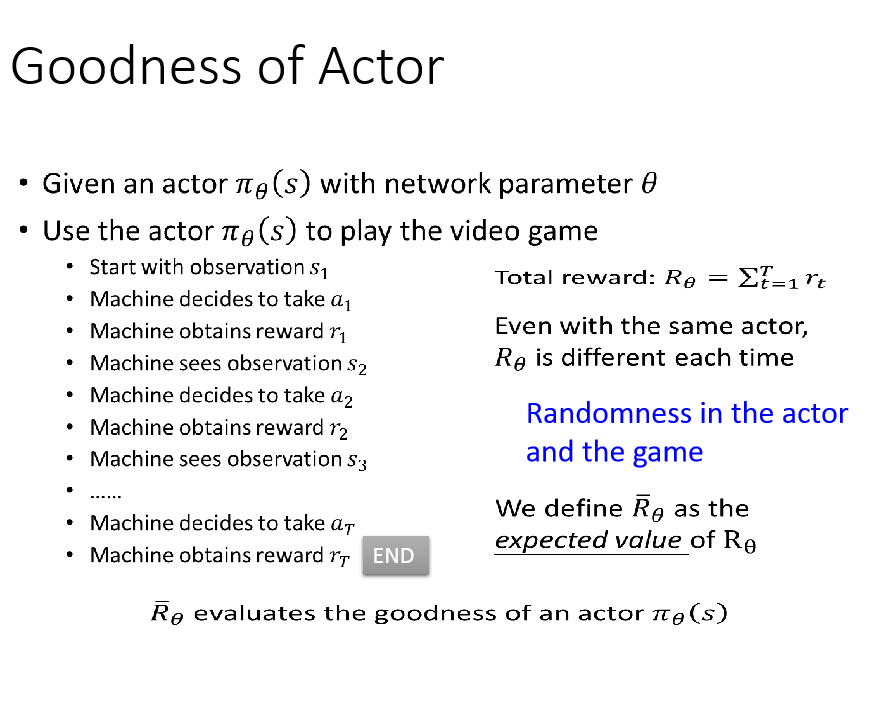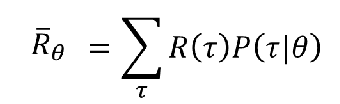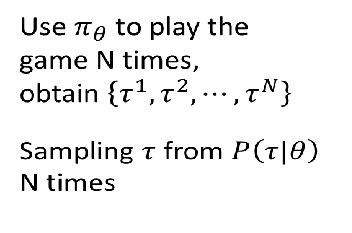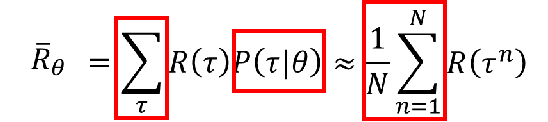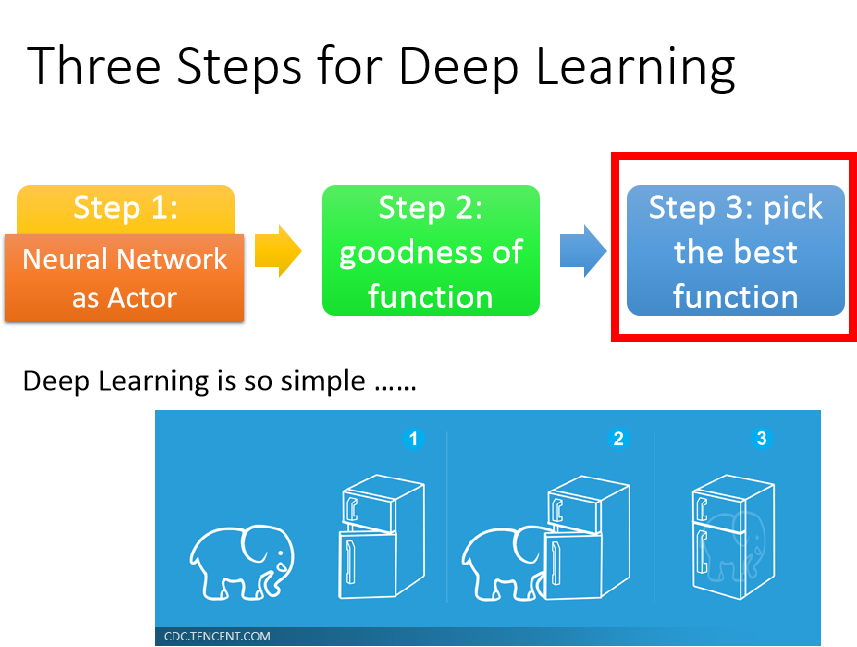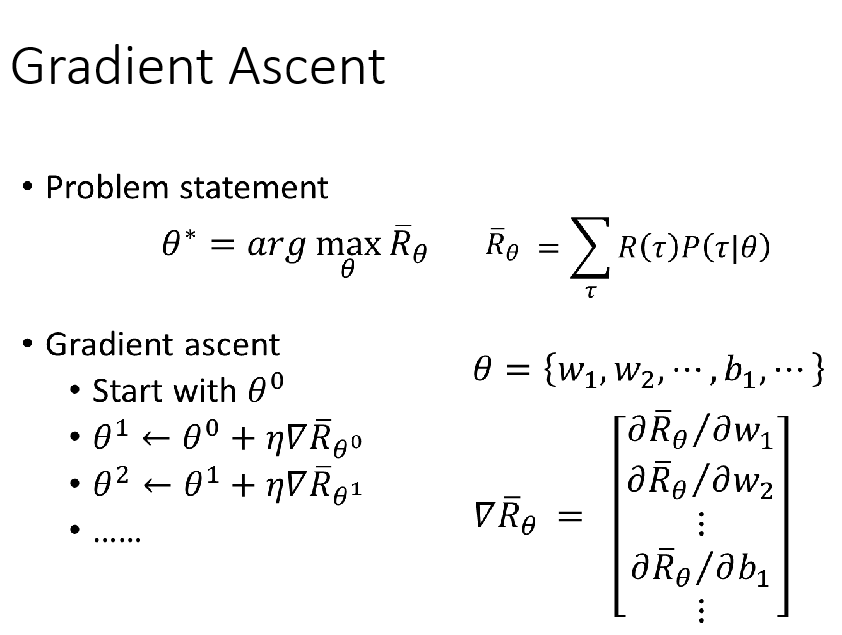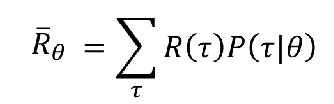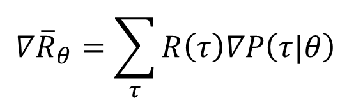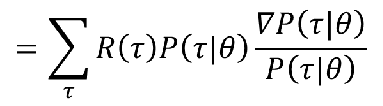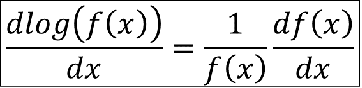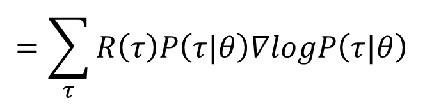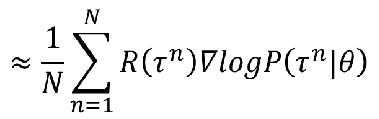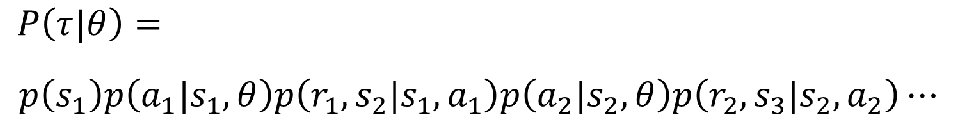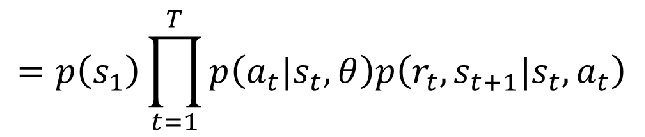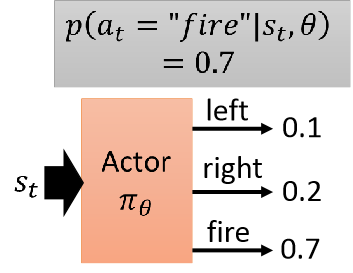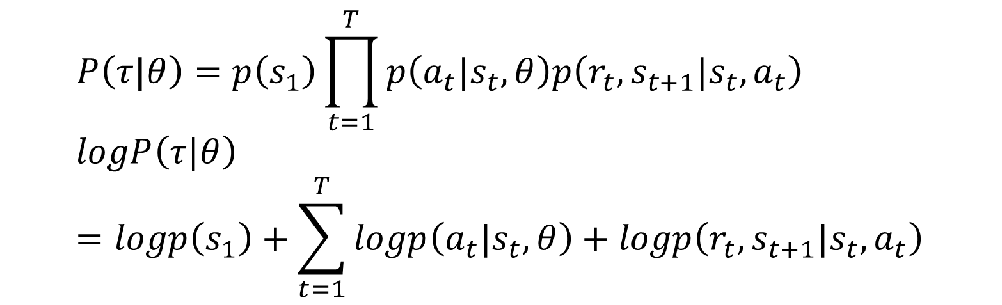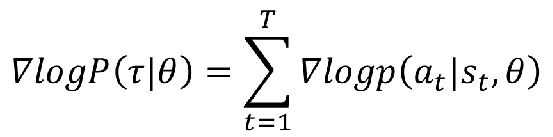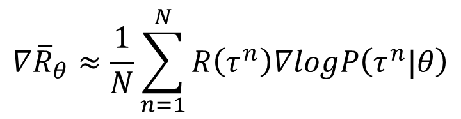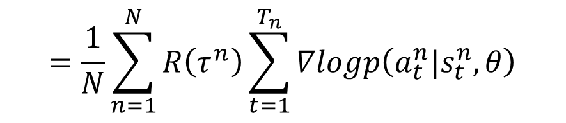$R(\tau^n)$作用于每一个梯度，所以可以乘进去，得：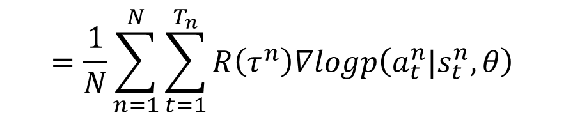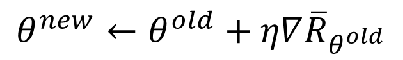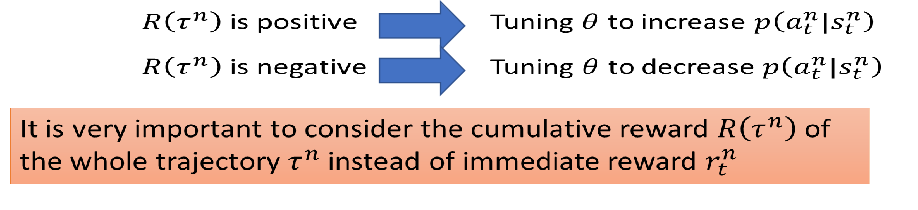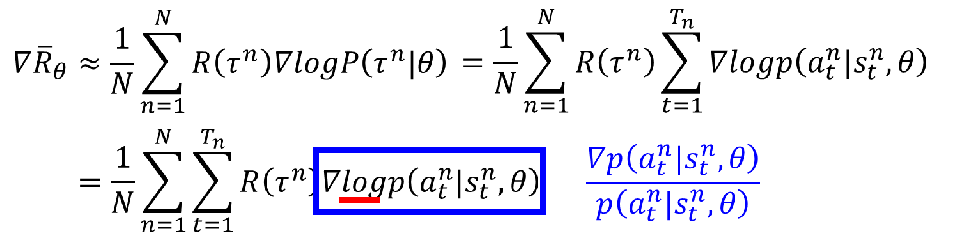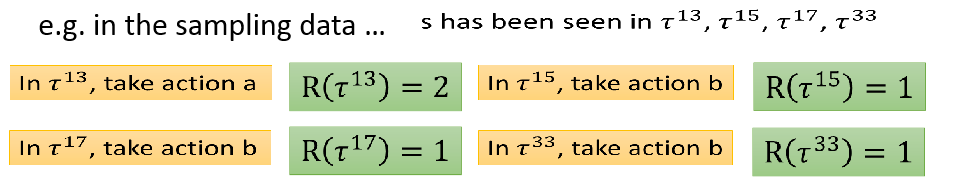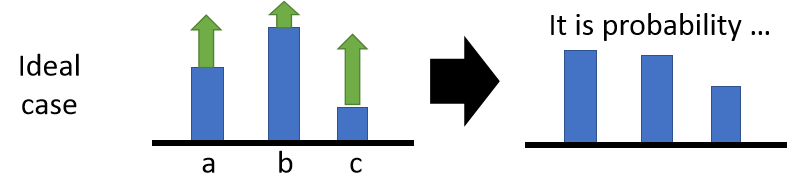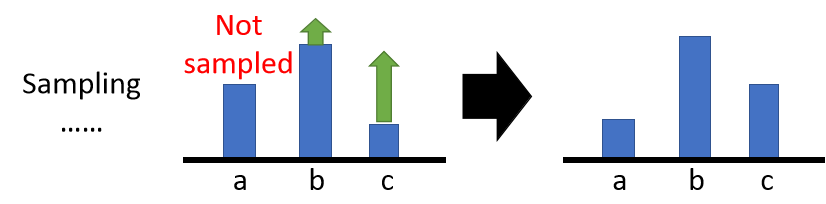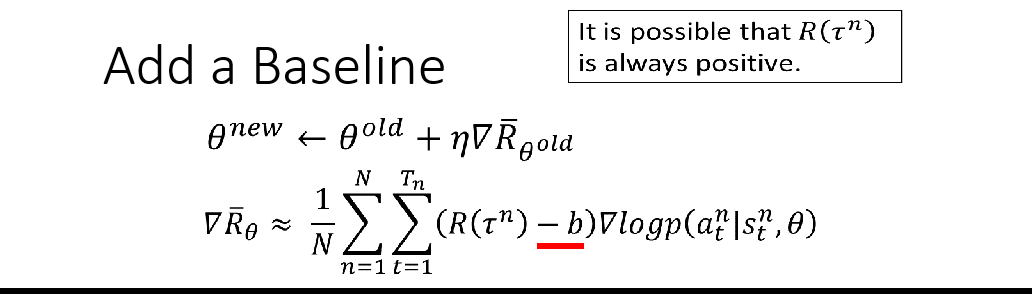# 总结

2017-09-30 17:22:05 jason_cuijiahui 阅读数 613
• ###### 深度学习基础与TensorFlow实践教程

本课程主要介绍了深度学习的基础原理和TensorFlow系统基本使用方法。TensorFlow是目前机器学习、深度学习领域优秀的计算系统之一，本课程将结合实例介绍使用TensorFlow开发机器学习应用的详细方法和步骤，着重讲解了用于图像识别的卷积神经网络和用于自然语言处理的循环神经网络的理论知识及其TensorFlow实现方法，并结合实际场景和例子描述了深度学习技术的应用范围与效果。 所有案例均来自讲师团队工作中的亲身实践，所选案例均是深度学习的经典应用，非常具有代表性。

21447 人正在学习 去看看 AI100讲师

## 摇杠赌博机的例子

2018-09-30 16:25:03 weixin_43092451 阅读数 241
• ###### 深度学习基础与TensorFlow实践教程

本课程主要介绍了深度学习的基础原理和TensorFlow系统基本使用方法。TensorFlow是目前机器学习、深度学习领域优秀的计算系统之一，本课程将结合实例介绍使用TensorFlow开发机器学习应用的详细方法和步骤，着重讲解了用于图像识别的卷积神经网络和用于自然语言处理的循环神经网络的理论知识及其TensorFlow实现方法，并结合实际场景和例子描述了深度学习技术的应用范围与效果。 所有案例均来自讲师团队工作中的亲身实践，所选案例均是深度学习的经典应用，非常具有代表性。

21447 人正在学习 去看看 AI100讲师1、机器人

2、工业自动化

3、加强预测性维护

4、游戏

2018-08-08 17:32:07 hzq20081121107 阅读数 267
• ###### 深度学习基础与TensorFlow实践教程

本课程主要介绍了深度学习的基础原理和TensorFlow系统基本使用方法。TensorFlow是目前机器学习、深度学习领域优秀的计算系统之一，本课程将结合实例介绍使用TensorFlow开发机器学习应用的详细方法和步骤，着重讲解了用于图像识别的卷积神经网络和用于自然语言处理的循环神经网络的理论知识及其TensorFlow实现方法，并结合实际场景和例子描述了深度学习技术的应用范围与效果。 所有案例均来自讲师团队工作中的亲身实践，所选案例均是深度学习的经典应用，非常具有代表性。

21447 人正在学习 去看看 AI100讲师

flappy bird 为例子来讲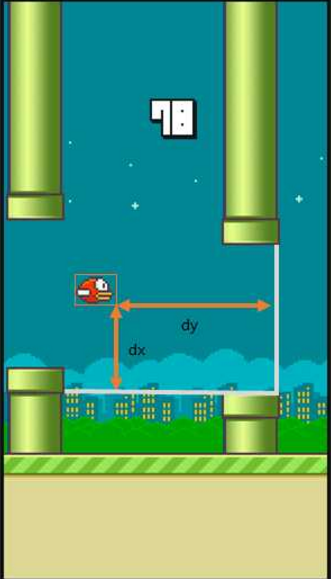https://www.zhihu.com/question/26408259

S：

d(x,y)表示小鸟离下一根柱子的距离和高度差

A：

Q（S+A->R）：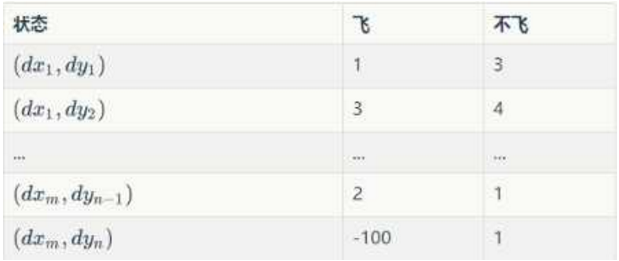Initialize Q arbitrarily //随机初始化Q值 (撞地上或者柱子上初始化为负值)

Repeat (for each episode): //每一次游戏，从小鸟出生到死亡是一个episode

Initialize S //小鸟刚开始飞，S为初始位置的状态

Repeat (for each step of episode):

。。。根据当前Q和位置S，使用一种策略，得到动作A //这个策略可以是ε-greedy等

做了动作A，小鸟到达新的位置S'，并获得奖励R //奖励可以是1，50或者-1000

Q(S,A) ← (1-α)*Q(S,A) + α*[R + γ*maxQ(S',a)] //在Q中更新S

S ← S'

until S is terminal //即到 小鸟死亡为止

Q(S,A) ← (1-α)*Q(S,A) + α*[R + γ*maxQ(S',a)]

1 当处于S状态采取A策略后，根据S'我们可以得到R, 那么这个R应该跟新到Q(S,A)中,我们可能会这样做：

Q(S,A) ← (1-α)*Q(S,A) + α*R

2 上面的公式太短视，我们更新Q(S,A) 时是不是要考虑一下，通过步骤A走到下一个状态S'的收益呢？

Q(S,A) ← (1-α)*Q(S,A) + α*[R + γ*Q(S',？)]

3 Q(S',？)表示状态S'的收益，Q(S',？)有飞和不飞2个值，我们既然知道奖惩，肯定是采用Q(S',？)中最大的那一种操作于是我们得到

Q(S,A) ← (1-α)*Q(S,A) + α*[R + γ*maxQ(S',a)]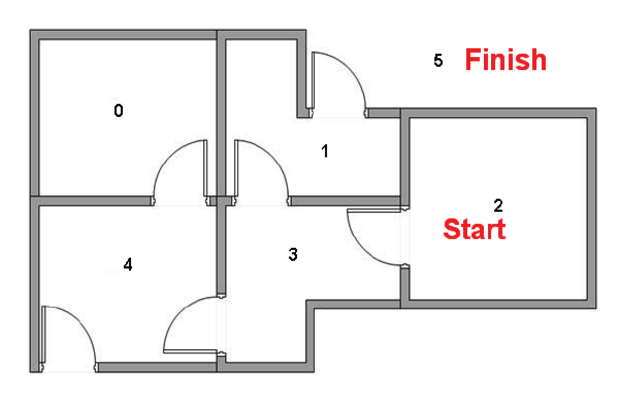#include<iostream>
#include<sstream>
#include<string>
#include<algorithm>
#include <stdlib.h>
#include <time.h>
#include<map>
using namespace std;
#define N 6
int pass[N][N];
float Q[N][N];
int dest;

void input()
{
int n,m,a,b,i,j;
//srand((unsigned)time(NULL));
for (i=0; i<N; i++)
{
for (j=0; j<N; j++){
pass[i][j] = 0;
Q[i][j] = 0;//(rand()%100)/100.0;//0;
}
}
cin>>n;//一共有n扇门
printf("%d doors\n",n);
while(n--){
cin>>a>>b;//房间a和b之间有互通
pass[a][b] = pass[b][a] = 1;
Q[a][b] = (rand()%100)/1000.0;//0;
Q[b][a] = (rand()%100)/1000.0;//0;
}
printf("input done\n");
//输入目标房间
cin>>m;//m为目的房间号
Q[m][m] = 1;//目标房间号的收益是最高的，为1
dest = m;
}

void displayQ(){
printf("\n");
int i,j;
for (i = 0; i < N; i++){
for (j = 0; j < N; j++){
printf("%.3f ",Q[i][j]);
}
printf("\n");
}
}
//从pqm中随机选出一个元素
int select_rand_ele(map <int, float> pqm){
int n = rand()%(pqm.size());
map<int, float>::iterator it = pqm.begin();
while(n--){
it ++;
}
return it->first;
}

//从一个矩阵的第pos行找出最大值，返回其位置
int find_max_Q(int pos, float &chosen_q){
int i;
int chosen_p = -1;
for (i=0; i<N; i++){
if (pass[pos][i] != 0){
if (chosen_p == -1 || Q[pos][i] > chosen_q)
{
chosen_q = Q[pos][i];
chosen_p = i;
}
}
}
return chosen_p;
}

//起始位置在序号为pos的房间，以0.3的概率走随机门
void train(int pos, float rr = 0.3){
printf("%d ", pos);
int i,j,k;
if (pos == dest){
return;
}
//action choice，开门换房
map <int, float> pqm;
float chosen_q;
int chosen_p = -1;
for (i=0; i<N; i++){
if (pass[pos][i] != 0){
pqm[i] = Q[pos][i];
if (chosen_p == -1 || Q[pos][i] > chosen_q)
{
chosen_q = Q[pos][i];
chosen_p = i;
}
}
}
if (chosen_p == -1){//这是一个没有门的房间
return;
}
//以一定概率走随机门
if ((rand()%100)/100.0 < rr){
chosen_p = select_rand_ele(pqm);
chosen_q = pqm[chosen_p];
}
// 更新Q
float lr = 0.5;// 学习率
float next_q_max_value = 0;
find_max_Q(chosen_p, next_q_max_value);
Q[pos][chosen_p] = lr * Q[pos][chosen_p] + (1-lr) *(next_q_max_value - 0.1);//走一扇门的代价是-0.1
//下一个位置
train(chosen_p, 0.3);
}

/*

6
4 5
4 3
3 2
1 3
0 4
1 5
5

6
4 5
4 3
3 2
1 3
0 4
1 5
2
*/
int main()
{
input();
displayQ();
int n = 1000;
while(n--){
train(rand()%N, 0.5);//从随机的一个房间开始走
displayQ();
}
system("pause");
}

2018-11-03 20:44:46 xckkcxxck 阅读数 503
• ###### 深度学习基础与TensorFlow实践教程

本课程主要介绍了深度学习的基础原理和TensorFlow系统基本使用方法。TensorFlow是目前机器学习、深度学习领域优秀的计算系统之一，本课程将结合实例介绍使用TensorFlow开发机器学习应用的详细方法和步骤，着重讲解了用于图像识别的卷积神经网络和用于自然语言处理的循环神经网络的理论知识及其TensorFlow实现方法，并结合实际场景和例子描述了深度学习技术的应用范围与效果。 所有案例均来自讲师团队工作中的亲身实践，所选案例均是深度学习的经典应用，非常具有代表性。

21447 人正在学习 去看看 AI100讲师

# -*- coding: utf-8 -*-
"""
Created on Mon Oct  1 22:20:10 2018

@author:
"""

import numpy as np
import pandas as pd
import time

np.random.seed(2)  # reproducible

N_STATES = 6   # 1维世界的宽度
ACTIONS = ['left', 'right']     # 探索者的可用动作
EPSILON = 0.9   # 贪婪度 greedy
ALPHA = 0.1     # 学习率
GAMMA = 0.9    # 奖励递减值
MAX_EPISODES = 13   # 最大回合数
FRESH_TIME = 0.3    # 移动间隔时间

#Q表：
def build_q_table(n_states, actions):
table = pd.DataFrame(
np.zeros((n_states, len(actions))),     # q_table 全 0 初始
columns=actions,    # columns 对应的是行为名称
)
return table

# 在某个 state 地点, 选择行为
def choose_action(state, q_table):
state_actions = q_table.iloc[state, :]  # 选出这个 state 的所有 action 值
if (np.random.uniform() > EPSILON) or (state_actions.all() == 0):  # 非贪婪 or 或者这个 state 还没有探索过
action_name = np.random.choice(ACTIONS)
else:
action_name = state_actions.argmax()    # 贪婪模式
return action_name

#环境反馈S_，R
def get_env_feedback(S, A):
# This is how agent will interact with the environment
if A == 'right':    # move right
if S == N_STATES - 2:   # terminate
S_ = 'terminal'
R = 1
else:
S_ = S + 1  #右移
R = 0
else:   # move left
R = 0
if S == 0:
S_ = S  # reach the wall
else:
S_ = S - 1 #左移
return S_, R

#环境更新
def update_env(S, episode, step_counter):
# This is how environment be updated
env_list = ['-']*(N_STATES-1) + ['T']   # '---------T' our environment
if S == 'terminal':
interaction = 'Episode %s: total_steps = %s' % (episode+1, step_counter)
print('\r{}'.format(interaction))
time.sleep(2)
print('\r                                ', end='')
else:
env_list[S] = 'o'
interaction = ''.join(env_list)
print('\r{}'.format(interaction), end='')
time.sleep(FRESH_TIME)

#强化学习主循环
def rl():
q_table = build_q_table(N_STATES, ACTIONS)  # 初始 q table
for episode in range(MAX_EPISODES):     # 回合
step_counter = 0
S = 0   # 回合初始位置
is_terminated = False   # 是否回合结束
update_env(S, episode, step_counter)    # 环境更新
while not is_terminated:

A = choose_action(S, q_table)   # 选行为
S_, R = get_env_feedback(S, A)  # 实施行为并得到环境的反馈
q_predict = q_table.loc[S, A]    # 估算的(状态-行为)值
if S_ != 'terminal':
q_target = R + GAMMA * q_table.iloc[S_, :].max()   #  实际的(状态-行为)值 (回合没结束)
else:
q_target = R     #  实际的(状态-行为)值 (回合结束)
is_terminated = True    # terminate this episode

q_table.loc[S, A] += ALPHA * (q_target - q_predict)  #  q_table 更新
S = S_  # 探索者移动到下一个 state

update_env(S, episode, step_counter+1)  # 环境更新

step_counter += 1
return q_table

if __name__ == "__main__":
q_table = rl()
print('\r\nQ-table:\n')
print(q_table)